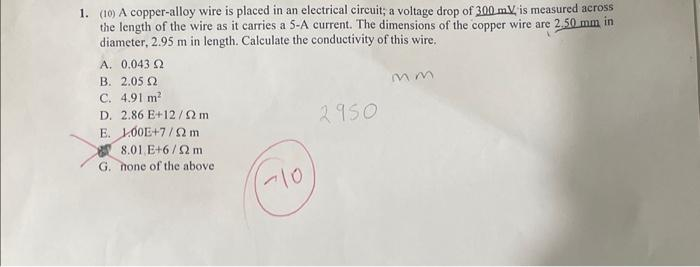Home / Expert Answers / Mechanical Engineering / 1-10-a-copper-alloy-wire-is-placed-in-an-electrical-circuit-a-voltage-drop-of-300mv-is-measure-pa761

# (Solved): 1. (10) A copper-alloy wire is placed in an electrical circuit; a voltage drop of 300mV, is measure ...1. (10) A copper-alloy wire is placed in an electrical circuit; a voltage drop of , is measured across the length of the wire as it carries a 5-A current. The dimensions of the copper wire are in diameter, in length. Calculate the conductivity of this wire. A. B. C. D. E. 8. G. none of the above

We have an Answer from Expert

Given data,
diameter of wire, = d = 2.50mm
Length of wire = l = 2.95 m
Current I, 5 A
Voltage drop,

Now we found resistance and cross sectional area

We have an Answer from Expert